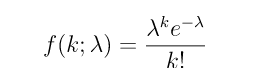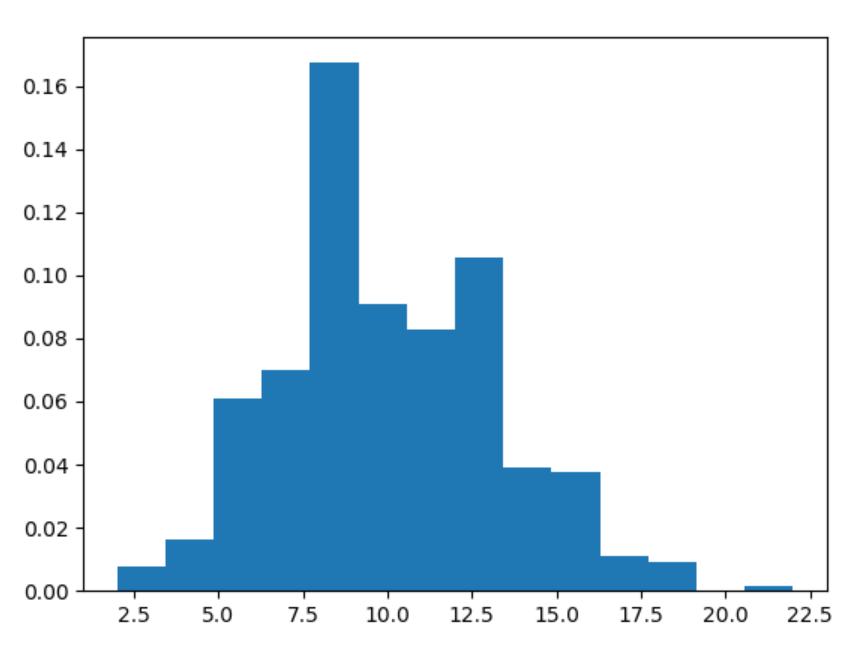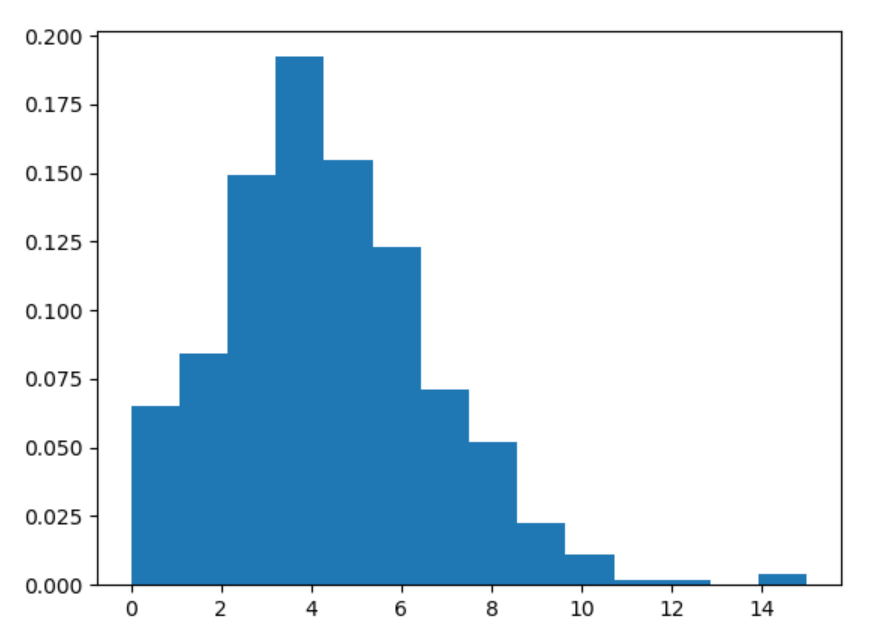# numpy.random.poisson() in Python

• Difficulty Level : Basic
• Last Updated : 15 Jul, 2020

With the help of numpy.random.poisson() method, we can get the random samples from poisson distribution and return the random samples by using this method.poisson distribution

Syntax : numpy.random.poisson(lam=1.0, size=None)

Return : Return the random samples as numpy array.

Example #1 :

In this example we can see that by using this numpy.random.poisson() method, we are able to get the random samples from poisson distribution by using this method.

## Python3

 `# import numpy``import` `numpy as np``import` `matplotlib.pyplot as plt`` ` `# Using poisson() method``gfg ``=` `np.random.poisson(``10``, ``1000``)`` ` `count, bins, ignored ``=` `plt.hist(gfg, ``14``, density ``=` `True``)``plt.show()`

Output :Example #2 :

## Python3

 `# import numpy``import` `numpy as np``import` `matplotlib.pyplot as plt`` ` `# Using poisson() method``gfg ``=` `np.random.poisson(``4.5``, ``500``)`` ` `count, bins, ignored ``=` `plt.hist(gfg, ``14``, density ``=` `True``)``plt.show()`

Output :My Personal Notes arrow_drop_up